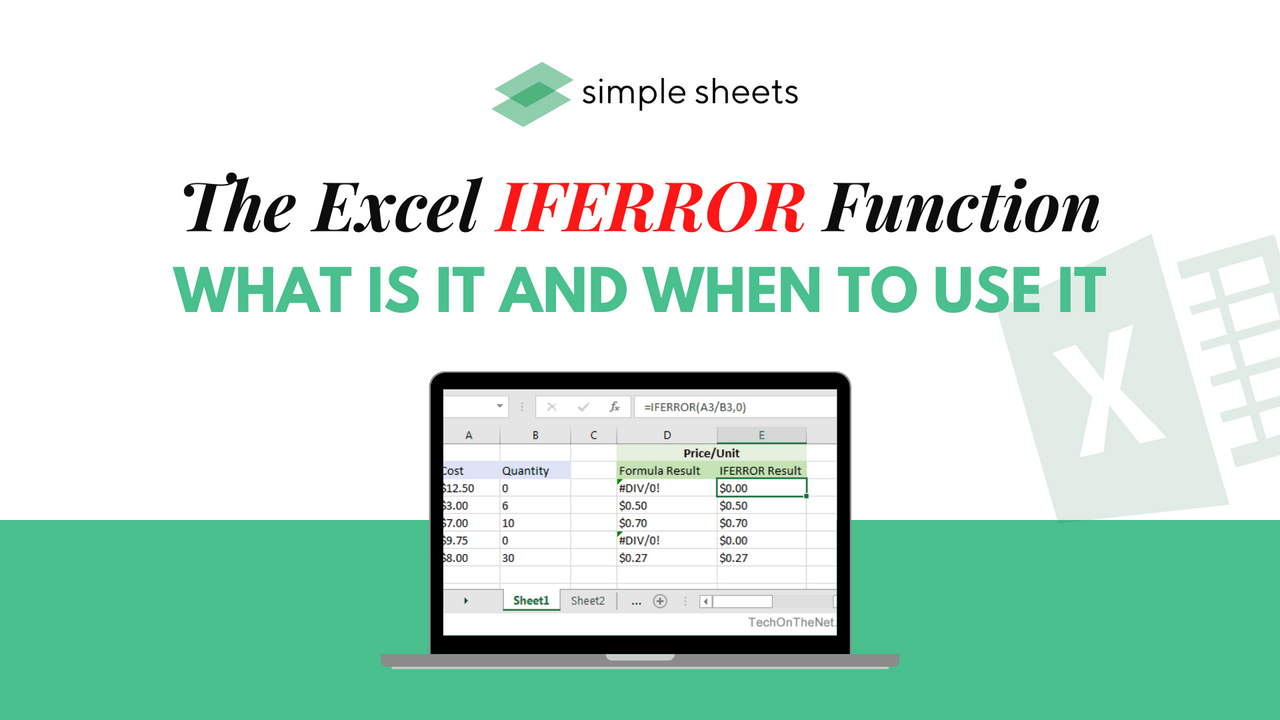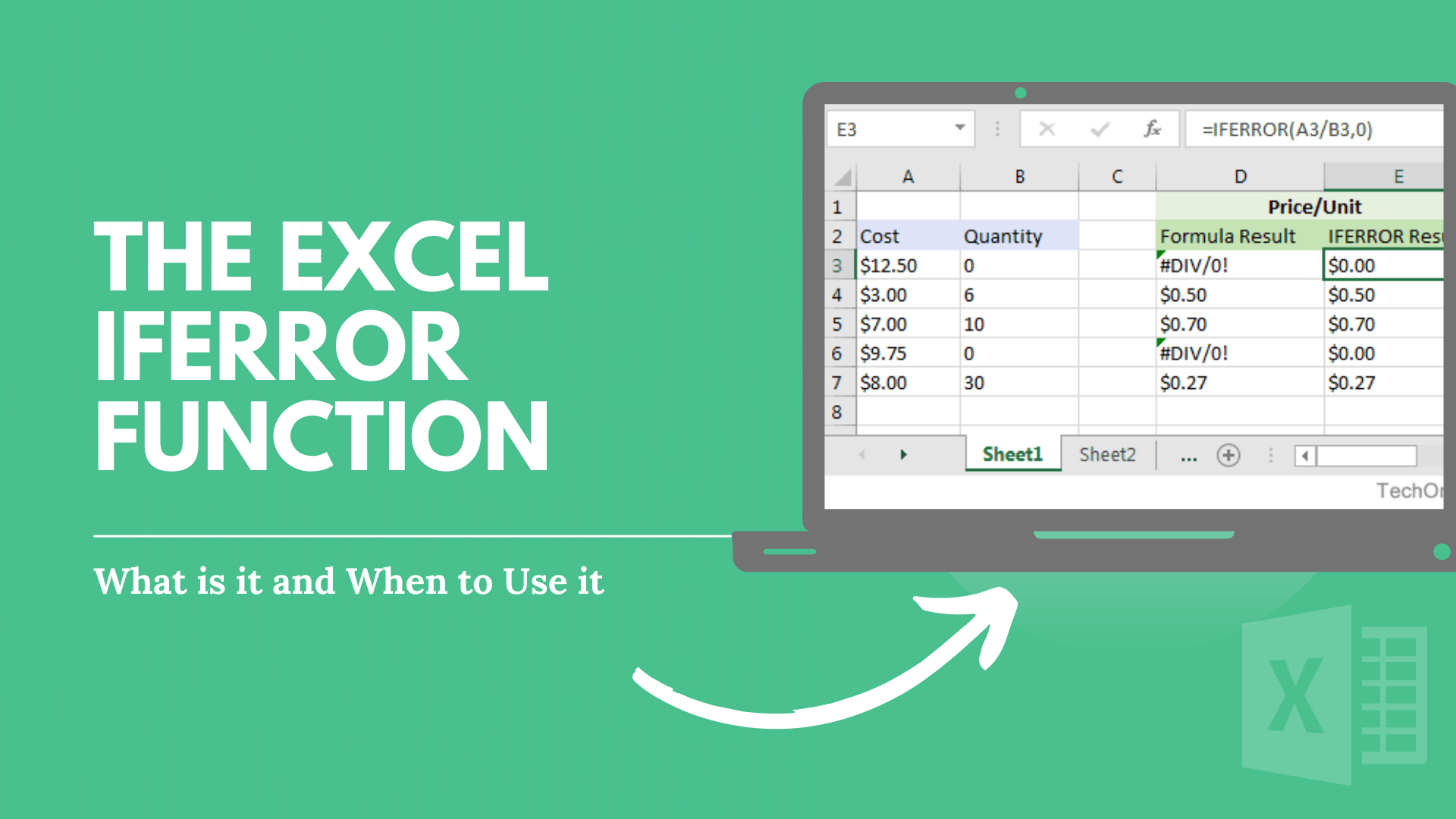# The Excel IFERROR Function: What is it and When to Use it

Jul 26, 2022If you have worked with Excel before, you have likely come across or heard of the Excel IFERROR function. It is one of the most useful Excel formulas at your disposal.

The IFERROR function within Microsoft Excel was built to manage any formula errors and calculation errors. IFERROR checks your formulas and identifies if there is an issue before returning the result of the formula. Here is the IFERROR syntax:

IFERROR(value, value_if_error) Value- this is the aspect of the formula that checks for errors. It might be an expression, a formula, a value, or a cell reference.

Value_if_error - this aspect is returned if an error occurs and is discovered. It might be an empty string value, a numeric value, another formula, or a text message.

For instance, if you divide two columns of numbers, you could end up with several errors if a column contains zeros, text, or empty cells. To prevent that, implement the IFERROR function to deal with errors.

This article will teach you everything you need to know about the Excel IFERROR function, Excel IFERROR function examples, and different Excel IFERROR function returns.## Things to Know About Excel IFERROR Function

• The Excel IFERROR function deals with all error types, such as #NULL!, #REF!, #DIV/0!, #NUM!, #VALUE!, #NAME?, and #N/A.
• IFERROR can replace errors with numeric customer values, dates, the result of other formulas, or text, depending on what the contents of the value_if_error aspect are.
• If your value argument is blank, it will be treated as an empty string, not an error.
• IFERROR function first appeared in Excel 2007 and has been available ever since.## IFERROR Formula Examples

The examples below will help you use the Excel IFERROR and other valuable functions for more complex tasks.

## Excel IFERROR with Vlookup

The Excel IFERROR function is most commonly used to tell users that a value they are looking for is not in the dataset.

To do this, wrapping a VLOOKUP function formula within the IFERROR function would be best. IFERROR(VLOOKUP(), "Not found") If your lookup value does not exist in your chosen table, a regular VLOOKUP formula will show a #N/A error.

## IFERROR in Array Formulas

As you are likely already aware, array formulas in Microsoft Excel are designed to execute multiple calculations in one formula.

If you give an array formula that results in an array of the value argument within an IFERROR function, you will get an array of values for every cell within your chosen range.## IFERROR vs. IF ISERROR

Now you understand how quick and easy it is to use the IFERROR function, and you might wonder why some people use the IF ISERROR combination. What are the advantages of this option? In truth, there aren't any!

In the past, IF ISERROR was the only way for users to trap errors. However, since Excel 2007, it has been a long and unnecessary process.## Best Ways to Use IFERROR in Excel

As you will know by now, the IFERROR function is a fantastic way to catch errors within Microsoft Excel and cover them with a custom message, a zero value, or a blank cell.

That said, you shouldn't wrap every formula with an error message. Here's how to keep the balance within your workbook.

1. Don't trap errors without reason.
2. Wrap as little as possible of the formula in IFERROR.
3. For specific errors, use one of these error-handling functions:
• ISERR, IFNA or IF ISNA

How do I use IFERROR in Excel?

The IFERROR function within Microsoft Excel was built to manage any formula errors and calculation errors. IFERROR checks your formulas and identifies if there is an issue before returning the result of the formula.

Can you use IFERROR and IF together?

You can use the ISERROR function, the ISERROR function, or the IFERROR function alongside IF.## Related Articles:

What is Apple Numbers For Mac: Everything You Need to Know

#### Want to Make Excel Work for You? Try out 5 Amazing Excel Templates & 5 Unique Lessons

We hate SPAM. We will never sell your information, for any reason.